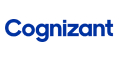New update is available. Click here to update.
Topics

# Candies

Moderate0/80
Average time to solve is 10m+10 more companies

## Problem statement

Prateek is a kindergarten teacher. He wants to give some candies to the children in his class. All the children stand in a line and each of them has a grade according to his or her performance in the class. Prateek wants to give at least one candy to each child. If two children are standing adjacent to each other, then the one with the higher rating must get more candies than the other. Prateek wants to minimize the total number of candies he must buy.

Given an array 'STUDENTS' of size 'N' that contains the grades for each student, your task is to find what is the minimum number of candies Prateek must buy so that he can distribute them among his students according to the criteria given above.

Example :

``````Given students' ratings : [5, 8, 1, 5, 9, 4].
He gives the students candy in the following minimal amounts : [1, 2, 1, 2, 3, 1]. He must buy a minimum of 10 candies.
``````

Note :

``````1. If two students having the same grade are standing next to each other, they may receive the same number of candies.
2. Every student must get at least a candy.
``````
Detailed explanation ( Input/output format, Notes, Images )
Constraints :
``````1 <= T <= 10^2
1 <= N <= 10^4
1 <= STUDENTS[i] <= 10^5

Time Limit : 1 sec
``````
Sample Input 1 :
``````3
2
1 5
3
1 3 4
3
1 2 2
``````
Sample Output 1 :
``````3
6
4
``````
Explanation For Sample Input 1 :
``````(i) Optimal distribution will be 1 2
(ii) Optimal distribution will be 1 2 3
(iii) Optimal distribution will be 1 2 1 because for children with equal grades one child can have less candies
``````
Sample Input 2 :
``````3
1
100
5
1 5 3 4 6
6
1 9 1 3 2 4
``````
Sample Output 2 :
``````1
9
9
``````
Explanation For Sample Input 2 :
``````(i) Optimal distribution will be 1
(ii) Optimal distribution will be 1 2 1 2 3
(iii) Optimal distribution will be 1 2 1 2 1 2
``````Console